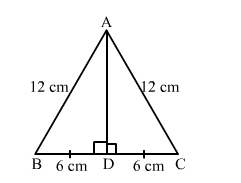# Find the height of an equilateral triangle of side 12 cm.

Question:

Find the height of an equilateral triangle of side 12 cm.

Solution:

Let ABC be the equilateral triangle with AD as an altitude from A meeting BC at D. Then, D will be the midpoint of BC.
Applying Pythagoras theorem in right-angled triangle ABD, we get:$A B^{2}=A D^{2}+B D^{2}$

$\Rightarrow A D^{2}=12^{2}-6^{2} \quad\left(\because B D=\frac{1}{2} B C=6\right)$

$\Rightarrow A D^{2}=144-36=108$

$\Rightarrow A D=\sqrt{108}=6 \sqrt{3} \mathrm{~cm}$

Hence, the height of the given triangle is $6 \sqrt{3} \mathrm{~cm}$.